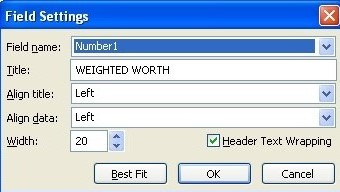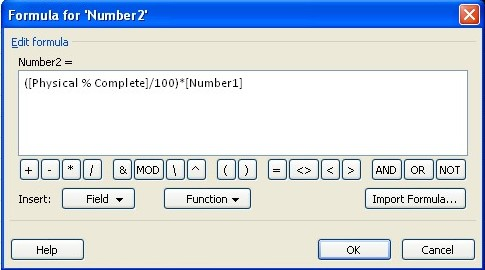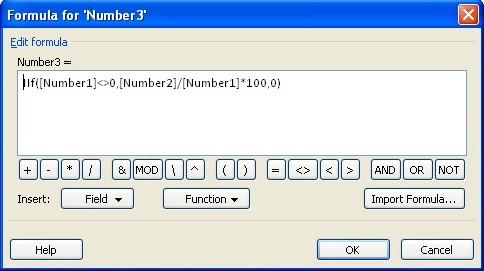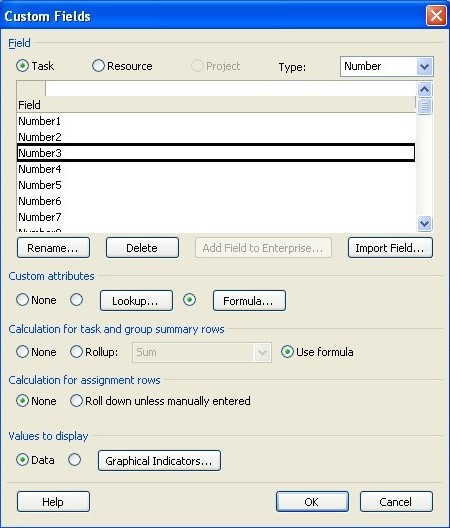# Updating the physical progress of the project with physical % complete

1. According to this, all the values ​​of this column are entered manually and the calculation of the summary of this field in the software is associated with problems, there is no principled and scientific formula for its calculation.
2. To solve the problem mentioned in row 1, we act as follows.
3. Define a project with 3 activities and two-day times in the form of FS. The project start date is August 24, 2015. Enter %Complete and Physical %Complete columns.
4. Create three columns named number2, number3, number1.
5. Name their titles in order, “physically finished%”, “weighted worth”, “physically finished weight”.1. Weighted Value is the weighted values ​​of activities that are entered manually. Select Rull Up from Sum type.
2. The physically finished weight field is the product of the Physical % Complete value in the weighted value. To calculate this value, we need to write the following formula. For the above field, select Roll Up from Sum type.8. The physically finished% field recalculates the new physical progress based on the weighting done. To calculate this column, we divide the value in the physically finished weight column by the weighted value.1. Make the following settings for this field as shown below.1. Update the project on August 28, 2015.

The value calculated in this field provides a more reliable figure than %Complete for project managers and planners.### The most practical formulas of Primavera

#### گروه بندی مقالات

###### سایر مقالات تخصصی